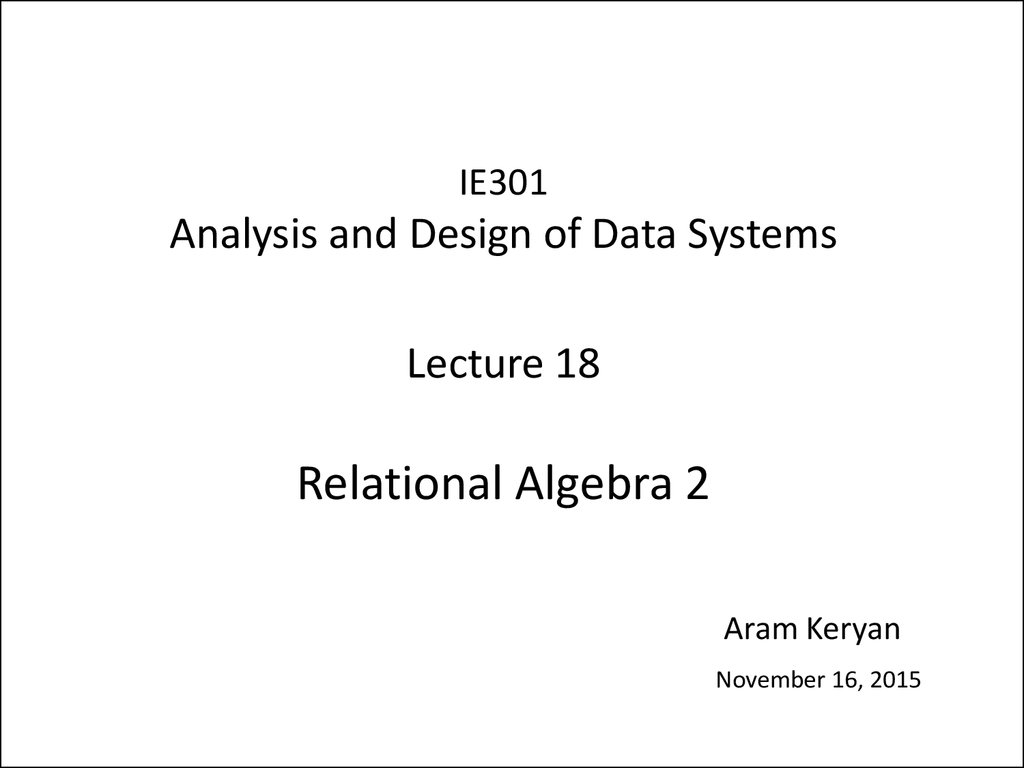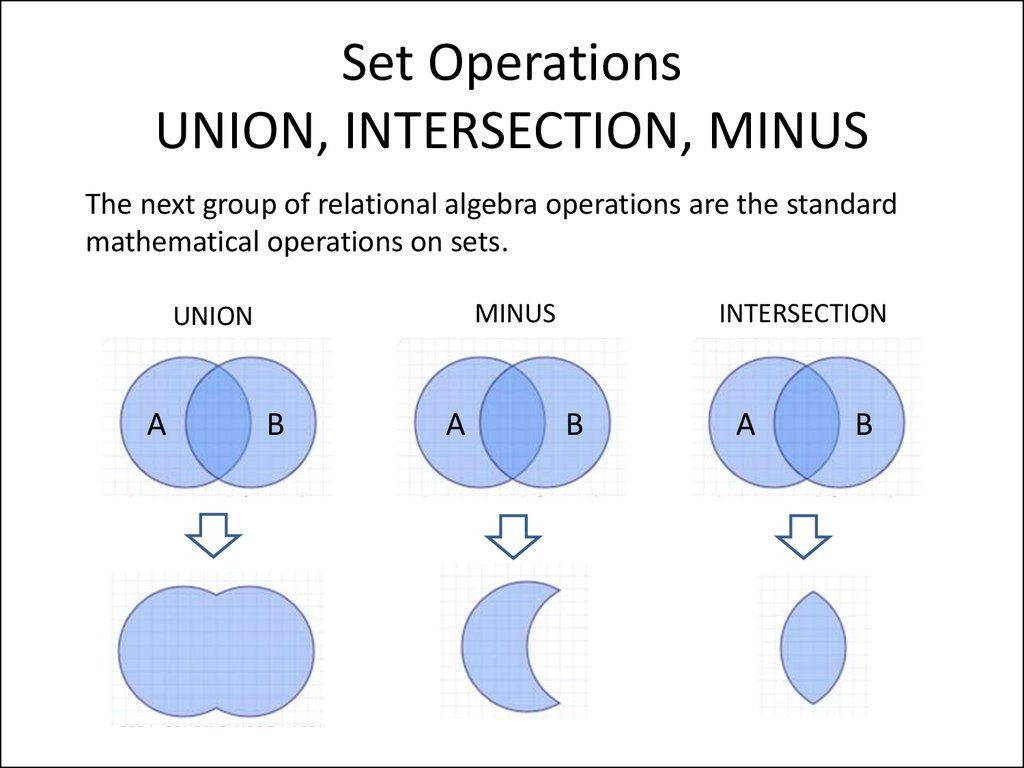# Analysis and Design of Data Systems. Relational Algebra 2 (Lecture 18)

## 1.

IE301
Analysis and Design of Data Systems
Lecture 18
Relational Algebra 2
Aram Keryan
November 16, 2015

## 2. Set Operations UNION, INTERSECTION, MINUS

The next group of relational algebra operations are the standard
mathematical operations on sets.
MINUS
UNION
A
B
A
INTERSECTION
B
A
B

## 3. Set Operations UNION, INTERSECTION, MINUS

UNION, INTERSECTION, and MINUS are binary operations.
Let’s